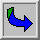Liste de Publications de Mathieu Colin.

• Publications dans des revues à comité de Lecture:

 D. Bresch, M. Colin, K. Msheik, P. Noble, X. Song, "Lubrication and shallow-water systems, Bernis-Friedman and BD entropies", Sousmis .pdf

 M. Colin and T. Iguchi, "Solitary wave solutions to the Isobe-Kakinuma model for water waves", Studies in Applied Mathematics, vol. 145(1), (2020), 52-80 .pdf

 M. Colin and T. Watanabe, "A refined stability result for standing waves of the Schrodinger-Maxwell system", Nonlinearity, vol. 32, (2019), 3695-3714 .pdf

 M. Colin and T. Watanabe, "Cauchy problem for the nonlinear Schrodinger equation coupled with the Maxwell equation", To appear in Annale Henri Lebesgue .pdf

  D. Bresch, M. Colin, K. Msheik, P. Noble and X. Song, "BD Entropy and Bernis-Friedman Entropy",C. R. Acad. Sci. Paris, Ser. I 357, (2019), 1-6 .pdf

 M. Colin and T. Watanabe, "On the existence of Ground states for a nonlinear Klein-Gordon-Maxwell type system", Funkcialaj Ekvacioj, vol. 61, (2018), 1-14 .pdf

 M. Colin and T. Watanabe, "Standing waves for the nonlinear Schrodinger equation coupled with the Maxwell equation", Nonlinearity, vol. 30 (5), (2017), 1920-1947 .pdf

 S. Bellec, M. Colin and M. Ricchuito, "Discrete asymptotic equations for long wave propagation", SIAM Journal on Numerical Analysis, vol.54 (6), (2016), 3280-3299 .pdf

 M. Colin and T. Watanabe, "Cauchy problem for the nonlinear Klein-Gordon equation coupled with the Maxwell equation", Journal of Mathematical Analysis and Application, vol.2, (2016), 778-796. .pdf

  M. Colin and S. Bellec. "On the existence of solitary waves for Boussinesq type equations and a new conservative model". Advances in Differential Equations, vol. 21 (9/10), (2016), 945-976., .pdf

 M. Colin, L. di Menza et J.-C.Saut, "Solitons in quadratic media". Nonlinearity, vol. 29 (3), (2016), 1000-1035. .pdf

 M. Colin, T. Colin and J. Dambrine. "Numerical simulation of wormlike micelle flows in micro-fluidic T-shaped junctions". Mathematics and Computer in Simulations, vol. 127, (2016), 28-55. .pdf

 A. Filipini, S. Bellec, M. Colin and M. Ricchuito. "On the nonlinear behavior of Boussinesq type-models : amplitude-velocity vs amplitude-flux forms". Costal Engineering, vol. 99, (2015), 109-123. .pdf

 M. Colin and M. Ohta. "Instability of ground states for a quasilinear Schrodinger equation". Diff. Int. Eqs., vol. 27 (7,8), (2014), 613-624.

 M. Colin and M. Ohta, "Bifurcation from semi-trivial standing waves and ground states for a system of nonlinear Schrodinger equations" SIAM Journal on Math. anal., vol. 44, (2012), 206-223

 M. Colin, T. Colin et J. Dambrine, "Validity of the Reynolds equation for miscible fluids in microchanneles" DCDS-B, vol. 17, (2012), 801-834. .pdf

 M. Colin et T. Colin, "A multi-D model for Raman amplification" M2AN, vol. 1, (2011), 1-22. .pdf

 M. Colin, L. Jeanjean et M. Squassina, "Stability and instability results for standing waves of quasi-linear Schrodinger equations" Nonlinearity, vol. 23(6), (2010),1353-1385.

 M. Colin et P. Fabrie, A variational approach for optimal control of the Navier-Stokes equations, Adv. Diff. Eqs. vol. 15, (2010), 829-852.

 M. Colin, T. Colin et M. Ohta, "Instability of standing waves for a system of nonlinear Schrodinger equations with three wave interaction" Funkcialaj Ekvacioj, vol. 52, (2009), 371-380.

 M. Colin, T. Colin et M. Ohta, "Stability of solitary waves for a system of nonlinear Schrodinger equations with three wave interaction", Ann. IHP, Analyse Non lineaire, vol. 26, (2009), 2211-2226.

 M. Colin et D. lannes, "Short pulses approximation in dispersive media", SIAM Journal on Mathematical Analysis, vol.41(2), (2009), 708-732. .pdf

 M. Colin and M. Ohta, "Stability of solitary waves for derivative nonlinear Schrödinger equation". Ann. IHP. Analyse Non Lineaire, vol. 23, (2006),

 M. Colin and T. Colin, "A numerical model for the Raman amplification for laser-plasma interaction". J. Comput. App. Math., vol. 193(2), (2006), 535-562.

 M. Colin and T. Colin, "On a quasilinear Zakharov system describing interactions laser-plasma". Diff. and Int. Eqs., vol. 17(3&4), (2004), 297-330

  M. Colin and L. Jeanjean, "Solutions for a quasilinear Schrödinger equation : a dual approach". Nonlinear Analysis, vol. 56(2), (2004), 213-226. .pdf

  M. Colin, "Approximation of a nonlinear Schrödinger equation by a Klein-Gordon equation". Asymptotic Analysis, vol. 34(3,4), (2003), 275-309 .pdf

  M. Colin, "Stability of stationary waves for a quasilinear Schrödinger equation in dimension 2" . Adv. in Diff. Eqs., vol. 8(1), (2003), 1-28 .

  M. Colin, "On the local well-posedness of quasilinear Schrödinger equations in arbitrary space dimension". Comm. Par. Diff. Eqs., vol. 27 (1&2), (2002), 325-354

• Actes de congrès- Autres :

 H. Kalisch, M. Ricchuito, P. Bonneton, M. Colin, P. Lubin, "Introduction to the special issue on breaking wave", European Journal of Mechanics B/Fluids, vol. 73, (2019), 1-5

 A. Filipini, S. Bellec, M. Colin and M. Ricchuito. "On shoaling properties of enhanced Boussinesq models". A paraitre dans ECMI 2014 Proceedings.

 J. Bona, M. Colin, T. Colin et D. Lannes, "Preface: special issue on asymptotic description of natural phenomena" DCDS, vol. 23, (2009), 41-06.

 M. Colin, T. Colin et G. Métivier, "Nonlinear models for laser-plasma interaction", Séminaire X-EDP 2006-2007. .pdf

 M. Colin et T. Colin, "Cauchy problem and numerical simulation for a quasilinear Zakharov system describing laser plasma interactions" Proceedings of the conference on nonlinear analysis, Orlando, Nonlinear Analysis, 63 (2005), e1679-e1686. .pdf

• Autres :

  "Etude mathématique d'équations de Schrödinger quasilinéaires intervenant en physique des plasmas". Mathieu COLIN, phD Thesis. .pdf

  "Etude de quelques problèmes issus de la physique des plasmas et de la mécanique des fluides". Mathieu COLIN, HDR, 2011. .pdf

• Mémoires :

[a]  "Etude d'un schéma Volume-Fini Boite". Mathieu COLIN, Mémoire de DEA sous la direction de J.P. Croisille.
[b]  "Les séries de Dirichlet". Mathieu COLIN, Mémoire de Magistère sous la direction de P. Boyer.Retour à la page principale.